Power Theorem Next  |  Prev  |  Up  |  Top  |  Index  |  JOS Index  |  JOS Pubs  |  JOS Home  |  Search

### Power Theorem

Theorem: For all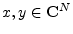,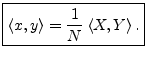Proof: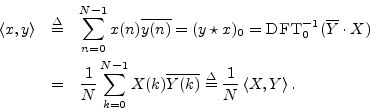Note that the power theorem would be more elegant if the DFT were defined as the coefficient of projection onto the normalized DFT sinusoid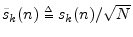. That, for the normalized DFT (defined in §6.10), the power theorem becomes simply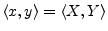(Normalized DFT case)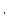The power theorem is also sometimes called Parseval's theorem .

In the field of physics, much progress has been made by formulating physical laws such that they are invariant with respect to changes of coordinates. The inner product is an example of a coordinate-invariant relationship between two vectors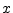and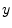in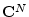. In the present setting, as developed in Chapter 5, we are concerned only with two different coordinate systems, corresponding to time-domain signals and their spectra.

Next  |  Prev  |  Up  |  Top  |  Index  |  JOS Index  |  JOS Pubs  |  JOS Home  |  Search

[How to cite this work] [Order a printed hardcopy]